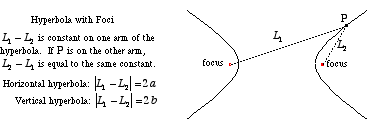index: click on a letter A B C D E F G H I J K L M N O P Q R S T U V W X Y Z A to Z index index: subject areas numbers & symbols sets, logic, proofs geometry algebra trigonometry advanced algebra & pre-calculus calculus advanced topics probability & statistics real world applications multimedia entrieswww.mathwords.com about mathwords website feedback

 Foci of a Hyperbola Two fixed points located inside each curve of a hyperbola that are used in the curve's formal definition. A hyperbola is defined as follows: For two given points, the foci, a hyperbola is the locus of points such that the difference between the distance to each focus is constant.See also